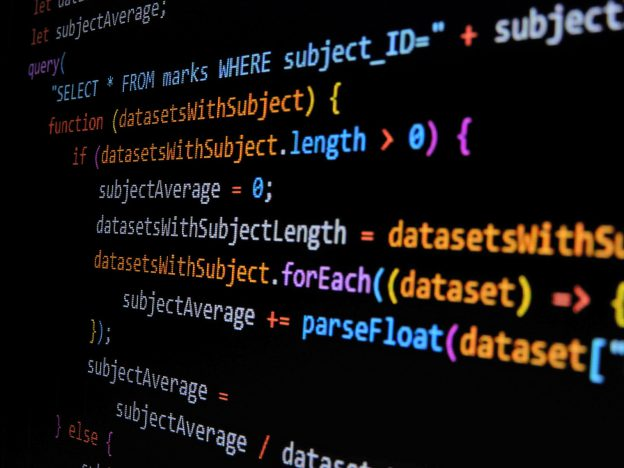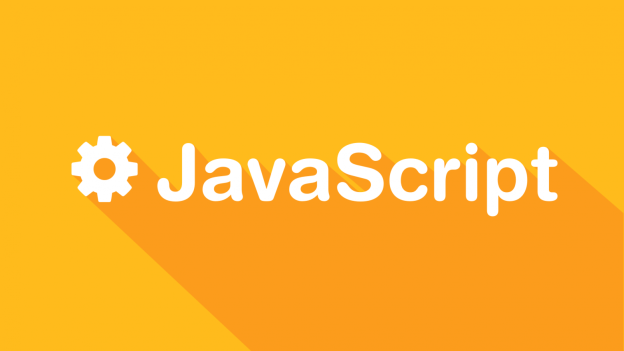# 标签归档：Javascript# warehouse — 一个简单易用的 JSON 数据库

warehouse 是一个 JSON 数据库，基于 JSON 实现了各种类似于 SQL 的查询，可以帮助我们基于一个 JSON 文件来进行查询。在 Hexo 的静态生成过程中，warehouse 帮了大忙。

### Example

``````var Database = require('warehouse');
var db = new Database();

var Post = db.model('posts', {
title: String,
created: {type: Date, default: Date.now}
});

Post.insert({
title: 'Hello world'
}).then(function(post){
console.log(post);
});``````

``````var db = new Database({
path: "./test.json", // 将数据存储在 test.json 当中
});
db.save();``````

``````var db = new Database({
path: "./test.json", // 将数据存储在 test.json 当中
});

## 场景# 在 Next.js 中直接引入 SVG

## 最简单的加载方式 —— URL 加载

``<img src="/logo.svg" alt="Logo SVG" />``

## 最舒服的加载方式 —— 编程加载

``````# yarn
# npm
npm install --save @svgr/webpack``````

``````module.exports = {
webpack(config) {
config.module.rules.push({
test: /\.svg\$/,
use: ["@svgr/webpack"]
}); // 针对 SVG 的处理规则

return config;
}
};``````

``````import Logo from "../assets/logo.svg";

// usage
<Logo />``````

## 总结# 如何解决 JavaScript 中 RegExp 不幂等的问题？

``````import type { NextApiRequest, NextApiResponse } from "next";
const URL = require("url");
const rule = /BV([0-9a-zA-Z]{1,10})/g;
export default function handler(
req: NextApiRequest,
res: NextApiResponse<MDResponse>
) {
const { url } = req.body;
const result = rule.exec(url);
}``````

result 的匹配结果不是每次都是可用的，会交替一次 null，一次有结果。

1. 在使用 Rule 之前，对其进行重置状态，即在进行匹配之前，先设定 rule.lastIndex 为 0
2. 另一种解决方案是将 rule 的定义放置在导出的函数内部，这样就可以在后续执行的过程中，重新初始化。

## Reference

https://stackoverflow.com/questions/38910334/regular-expression-exec-function-does-not-work-multiple-times

# 实现密码生成器的思路

``````function GeneratePassword() {
if (parseInt(navigator.appVersion) <= 3) {
alert("Sorry this only works in 4.0+ browsers");
return true;
}
var length=8;
length = document.aForm.charLen.options[document.aForm.charLen.selectedIndex].value;
var noPunction = (document.aForm.punc.checked);
var randomLength = (document.aForm.rLen.checked);
if (randomLength) {
length = Math.random();
length = parseInt(length * 100);
length = (length % 7) + 6
}
for (i=0; i < length; i++) {
numI = getRandomNum();
if (noPunction) { while (checkPunc(numI)) { numI = getRandomNum(); } }
}
return true;
}
function getRandomNum() {
// between 0 - 1
var rndNum = Math.random()
// rndNum from 0 - 1000
rndNum = parseInt(rndNum * 1000);
// rndNum from 33 - 127
rndNum = (rndNum % 94) + 33;
return rndNum;
}
function checkPunc(num) {
if ((num >=33) && (num <=47)) { return true; }
if ((num >=58) && (num <=64)) { return true; }
if ((num >=91) && (num <=96)) { return true; }
if ((num >=123) && (num <=126)) { return true; }
return false;
}
``````

checkPunc 则是检测输出的文字是否可以直接发音，这涉及到记忆的难度的问题，在一个长的字符串中，记忆 ABCD 肯定比记忆逗号、破折号、句号等符号要简单一些，毕竟字母肯定要好记忆点，可以自编语句来实现。

``````function GeneratePassword() {
// 检测浏览器支持
if (parseInt(navigator.appVersion) <= 3) {
alert("Sorry this only works in 4.0+ browsers");
return true;
}
var length=8;
length = 10 // 此处代码原为使用 dom 函数获取表单数据的代码，来实现自定义长度的功能。
var noPunction = false// 此处代码原为获取表单数据的代码，控制是否检测密码的可读性
var randomLength = false // 此处代码原为获取表单数据的代码，控制是否使用随机长度。
// 如果使用随机长度的密码，则进入循环
if (randomLength) {
length = Math.random();
length = parseInt(length * 100); // 生成 0 ~ 100 之间的数字
length = (length % 7) + 6 // 对生成的长度取余，则最短为6，最长为12
}
// 根据长度进行循环
for (i=0; i < length; i++) {
numI = getRandomNum();  // 获取一个随机数
if (noPunction) { while (checkPunc(numI)) { numI = getRandomNum(); } } // 如果需要判断发音，进行发音判断，判断失败就重新生成，直到成功。
}
// 设置代码到 Dom 元素中
return true;
}``````# 使用 JavaScript 来导出 CSV

``````var data = [
{
"title" :value,
"href" : value
},
...
]``````

``````function convertArrayOfObjectsToCSV(args) {
var result, ctr, keys, columnDelimiter, lineDelimiter, data;
data = args.data || null;
if (data == null || !data.length) {
return null;
}
columnDelimiter = args.columnDelimiter || ',';
lineDelimiter = args.lineDelimiter || '\n';
keys = Object.keys(data);
result = '';
result += keys.join(columnDelimiter);
result += lineDelimiter;
data.forEach(function(item) {
ctr = 0;
keys.forEach(function(key) {
if (ctr > 0) result += columnDelimiter;
result += item[key];
ctr++;
});
result += lineDelimiter;
});
return result;
}``````

``````function downloadCSV(args) {
var csv = convertArrayOfObjectsToCSV({
data: data
});
if (csv == null) return;
filename = args.filename || 'export.csv';
if (!csv.match(/^data:text\/csv/i)) {
csv = 'data:text/csv;charset=utf-8,' + csv;
}
data = encodeURI(csv);``var delay = 1000;function del() {    document.querySelector('.app_canvas_frame').contentDocument.querySelector('.del_btn').click();    setTimeout("yes()", delay);}function yes() {    document.querySelector('.qz_dialog_layer_btn').click();    setTimeout("del()", delay);}del();``